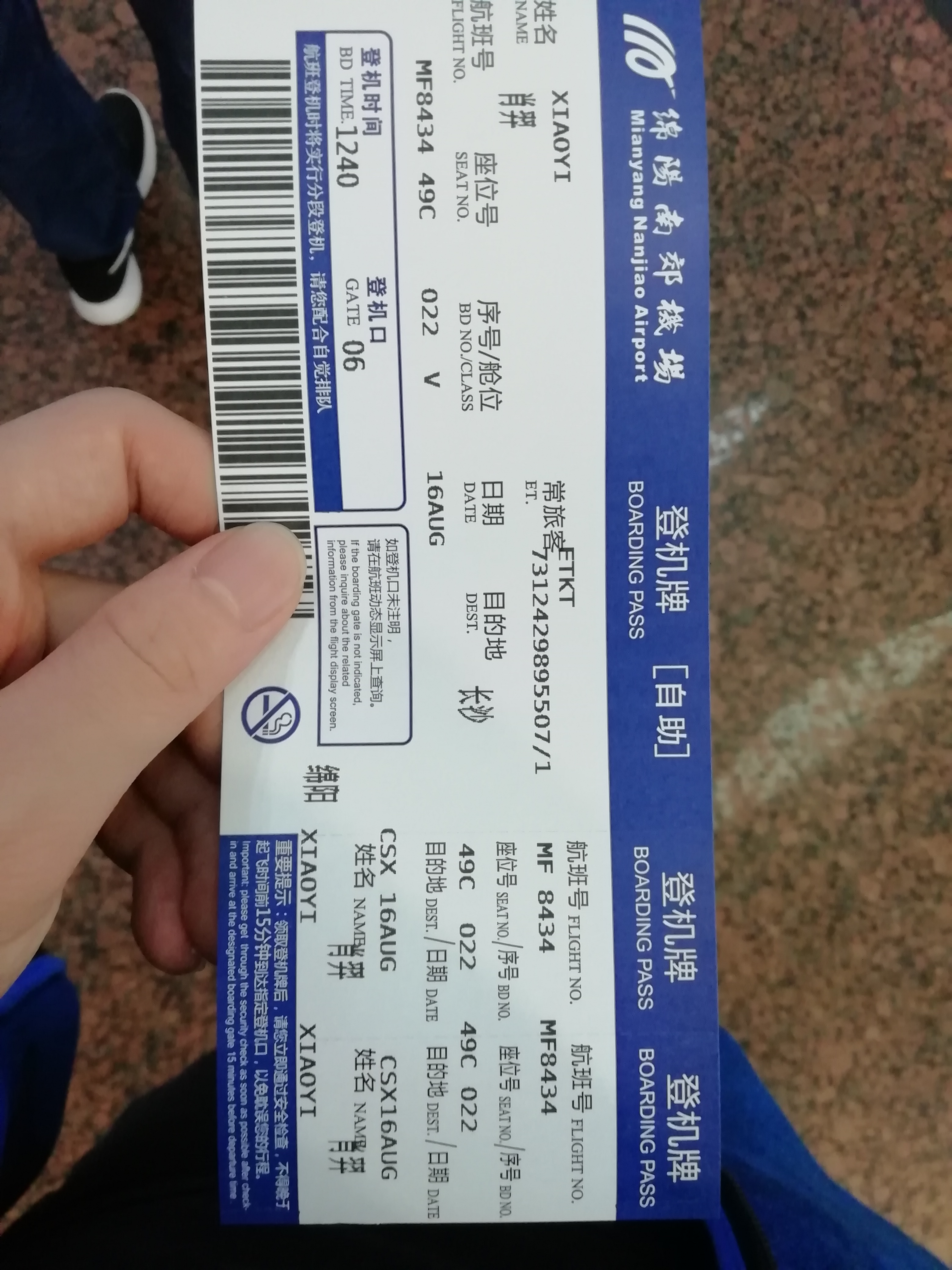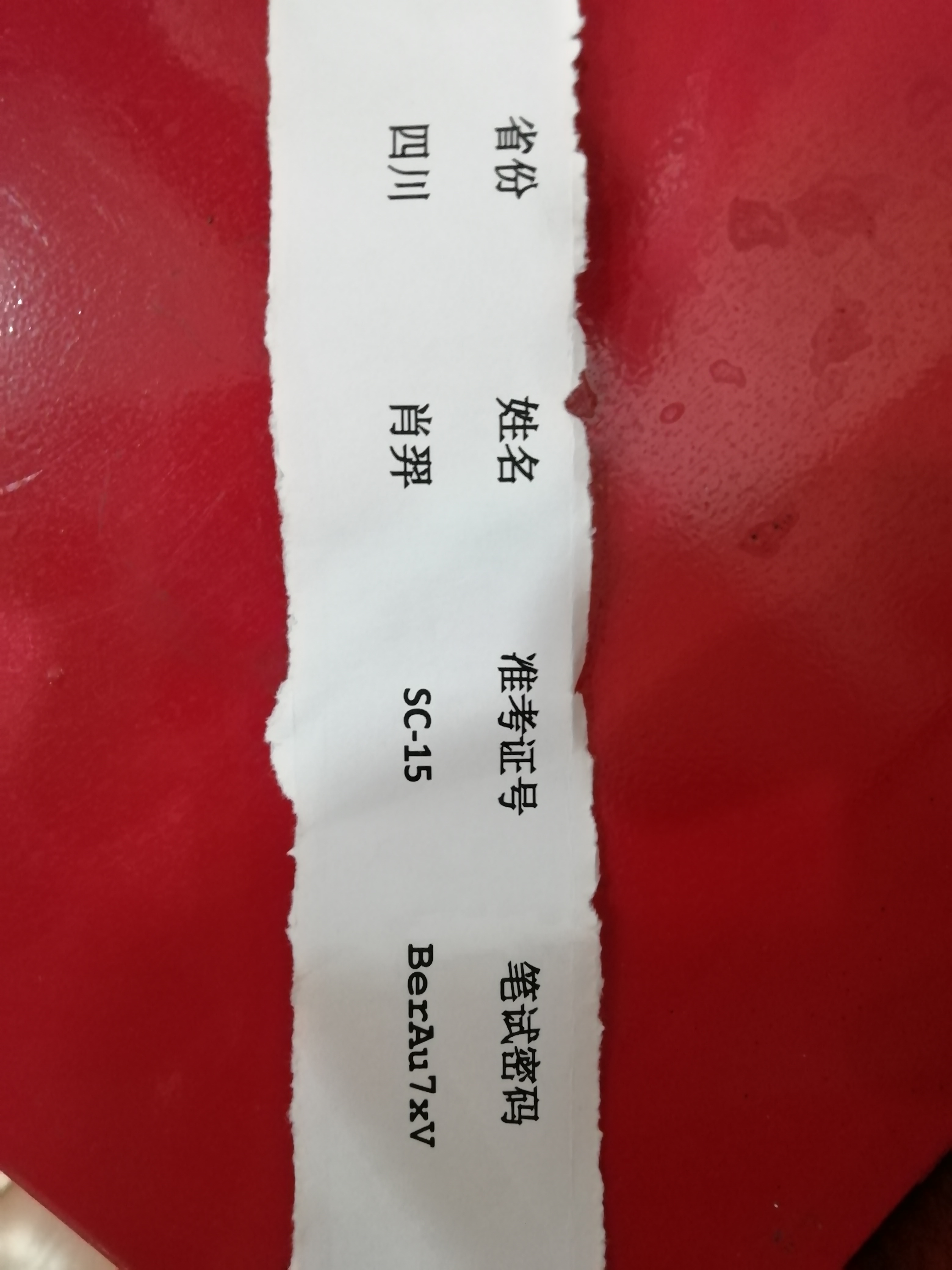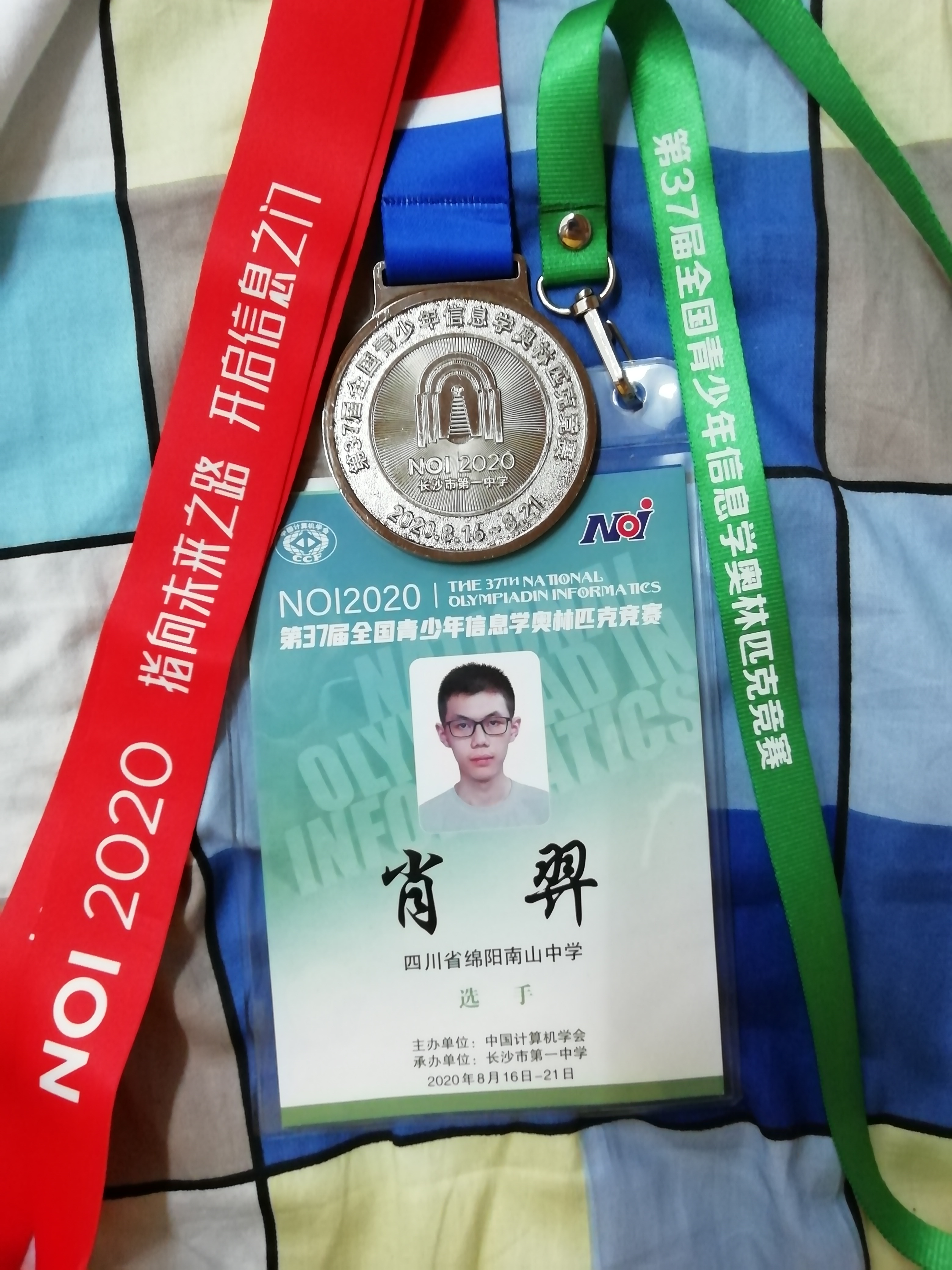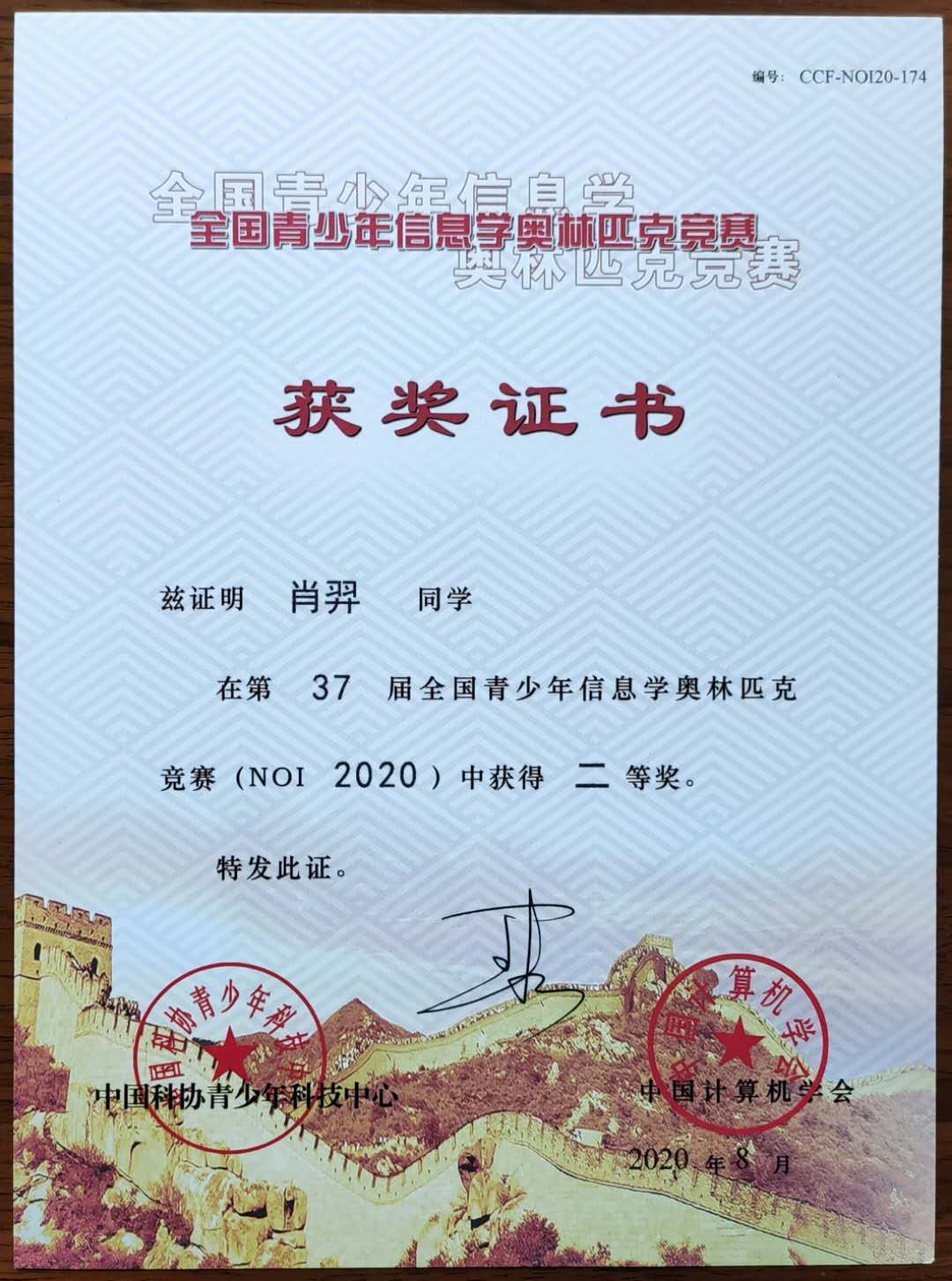# 【杂文】银色的 NOI2020（退役记）

$\color{red}\Large{{\mathcal{M}}\color{#fbe044}{\mathcal{y}}\ \ \color{green}{\mathcal{B}}\color{#46f1e7}{\mathcal{l}}\color{blue}{\mathcal{o}}\color{purple}{\mathcal{g}}}$

$\text{NOI}$ 的难度一年比一年高，而以我的思维水平连一道省选级别的数据结构都做不出来，难道考场上只能寄希望于死磕字符串套路码题？或许做出一道像你的名字那样的题能使我的分数不会太难看？

## 时间：2020-7-20

【禁則事項】 做了套 $\text{NOIP}$ 模拟题，结果 $\text{T2}$ 写挂、 $\text{T3}$ 没写完，被两个初中生吊锤几十分，颜面何存啊 o(╥﹏╥)o

## 时间：2020-8-9

（上一次简直差劲到了极点，甚至坏了一天的心情）

## 时间：2020-8-16 (day-1)——あさがおと加瀬さん

## 时间：2020-8-17 (day0)

（这密码条仿佛在暗示着什么 qaq）## 时间：2020-8-18 (day1)

$\text{T3}$ 特殊性质比较多，而且是序列上的问题扩展，嘤该比较好拿分，但保险起见还是决定按顺序开题。

$\text{T1}$ 稍想了会儿，发现是个矩阵弱智题，直接扩成 $w$ 倍暴力乘即可。常数略危险，用和 $\text{NOIO#3 T2}$ 一样的套路，预处理矩阵幂次，然后查询时用向量乘矩阵降维，复杂度 $O((wn)^3\log T+k(wn)^2\log T)$ 。一开始以为矩乘会很慢，于是只处理了 $10$ 次幂，但没想到前面快得飞起、后面慢得要死，于是改成 $29$，然后 $n=50,T=10^9,k=80$ 时（由大样例手动 $\text{rand}$ 搞出来的数据）只跑了 $0.5$，感觉比较稳。

$\text{T2}$ 枚举哪些路径不覆盖的情况，剩下的边随便选，得到至少 $x$ 条路径不选的方案数 $g(x)$，然后二项式反演得到恰好 $0$ 条边不选的方案数： $f(0)=\sum_{i=0}^{n}(-1)^{i}g(i)$ 。统计哪些没覆盖时可以树上差分维护，复杂度 $O(2^mn)$，能过 $24pts$ 。然后上树剖降到 $O(2^m\log^2 n)$，卡卡常似乎能过 $40pts$（有 $\text{O2}$ 就是可以为所欲为）。

$\text{T3}$ 果然是最好得分的，前 $24pts$ 直接模拟一下求逆序对就完事了，复杂度 $O(nm\log n)$ 。一维的莫队暴力搞，复杂度 $O((n+m)\sqrt{n}\log n)$ 。最后特殊性质 $\text{C}$ 就 $set$ 预处理出所有逆序对，然后主席树查询二维区间内点的个数，再暴力枚举减掉内部的逆序对即可，复杂度 $O((n+m)\log n)$ 。

$3$ 点看分。隔壁老哥自称来自弱省，说强省 $\text{SC}$ 都是巨佬，在一边疯狂奶我，结果点开一看发现前两道都挂了。 $\text{T1}$ 不知道哪儿出了问题， $\text{T2}$ 容斥时 $-1$ 没有加 $P$ 取模，答案炸成了负数。

$\text{tmd}$ 你前段时间那两个月的计数都白学了？连一个取模都能搞漏，考前颓废颓傻了？

## 时间：2020-8-19 (day2)

——你以为我会这么说吗？
......说不定还真会（这人疯了）。

$\text{T1}$ 想了下发现网络瘤大概只能用来骗分（还是最低档的），贪心构造似乎要靠谱一点，于是开始尝试骗分。

$\text{T2}$ 完全不会（暴力都不会打）。感觉天花板都快掉下来砸我头上了。
$\text{T3}$ 依旧不会。不过 $25pts$ 的性质 $B$ 似乎就是交叉着跑。写出来后发现大样例跑不出来，仔细一想才意识到有可能往回走，这样的话就得想办法跑 $dp$，但....总感觉非常麻烦，于是放弃了。

$\text{T1}$ 第二档 $n\leqslant 10$ 的部分居然都是人工构造的带有特殊性质的数据（即和大样例一样，都是只存在一条链，剩下的用一个大数刚好匹配）！

$\text{T2}$ 判挂了链的情况， $\text{T3}$ 那 $5pts$ 好像是是无解输出 $-1$ 。

$\text{pkh}$ 挂了点分，只有 $40$ 。

$\text{day2}$ 分数普遍偏低，难以拉开差距。

## 时间：2020-8-20 (day3)

（ $\text{zyy}$）
Q: 如果因为疫情原因彻底取消今年的 $\text{IOI}$，你有什么打算？
A: 学习大学的内容。

（ $\text{lyx}$）
Q: 还是和刚刚 $\text{zyy}$ 同学一样的问题。他现在高三，但你还是高二，你准备怎么办？（不要套用他的回答）
A: 虽然还是高二，但我报了清华的英才班，所以也会学习大学知识。

（ $\text{zrf}$） Q: 你现在是第 $5$ 名，相比于前面四位同学，你有什么优势？（给我一个换掉前四的理由）
A: 我学的早，现在也已经高三了，经历了风风雨雨，我的经历比他们绝大多数人都要丰富，我有着他们所不具备的强大心理素质。（我身经百战，我 $\text{nb}$，我 $\text{txdy}$！）
Q: 你的英语口语非常标准，普通话也非常流利，但你的教练徐先友说的是地道方言，请问你会受到影响吗？
A: 语言习惯会随环境而改变，但我周围说方言的只有他一人，同学们说的都是普通话，因此不会出现这种问题。